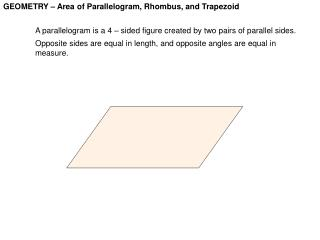# GEOMETRY – Area of Parallelogram, Rhombus, and Trapezoid - PowerPoint PPT PresentationDownload PresentationGEOMETRY – Area of Parallelogram, Rhombus, and Trapezoid

GEOMETRY – Area of Parallelogram, Rhombus, and TrapezoidDownload Presentation## GEOMETRY – Area of Parallelogram, Rhombus, and Trapezoid

- - - - - - - - - - - - - - - - - - - - - - - - - - - E N D - - - - - - - - - - - - - - - - - - - - - - - - - - -
##### Presentation Transcript

1. GEOMETRY – Area of Parallelogram, Rhombus, and Trapezoid A parallelogram is a 4 – sided figure created by two pairs of parallel sides. Opposite sides are equal in length, and opposite angles are equal in measure.

2. GEOMETRY – Area of Parallelogram, Rhombus, and Trapezoid A parallelogram is a 4 – sided figure created by two pairs of parallel sides. Opposite sides are equal in length, and opposite angles are equal in measure. When dealing with area, they are drawn with an altitude.

3. GEOMETRY – Area of Parallelogram, Rhombus, and Trapezoid A parallelogram is a 4 – sided figure created by two pairs of parallel sides. Opposite sides are equal in length, and opposite angles are equal in measure. When dealing with area, they are drawn with an altitude ( height ). height base

4. GEOMETRY – Area of Parallelogram, Rhombus, and Trapezoid EXAMPLE # 1 : Find the area of a parallelogram with base 12 and height of 5. height base

5. GEOMETRY – Area of Parallelogram, Rhombus, and Trapezoid EXAMPLE # 1 : Find the area of a parallelogram with base 12m and height of 5m. height base

6. GEOMETRY – Area of Parallelogram, Rhombus, and Trapezoid EXAMPLE # 2 : Find the area of a parallelogram with base 3.5 ft and height of 12.7 ft. height base

7. GEOMETRY – Area of Parallelogram, Rhombus, and Trapezoid EXAMPLE # 2 : Find the area of a parallelogram with base 3.5 ft and height of 12.7 ft. height base

8. GEOMETRY – Area of Parallelogram, Rhombus, and Trapezoid EXAMPLE # 3 : If the area of a parallelogram is 125 square inches, find the height if the base is 25 inches. height base

9. GEOMETRY – Area of Parallelogram, Rhombus, and Trapezoid A rhombus is a special parallelogram with all 4 sides equal.

10. GEOMETRY – Area of Parallelogram, Rhombus, and Trapezoid A rhombus is a special parallelogram with all 4 sides equal. Just like a parallelogram, it can be drawn with a height.

11. GEOMETRY – Area of Parallelogram, Rhombus, and Trapezoid A rhombus is a special parallelogram with all 4 sides equal. Just like a parallelogram, it can be drawn with a height. It can also be drawn with diagonals. d1 d2

12. GEOMETRY – Area of Parallelogram, Rhombus, and Trapezoid A rhombus is a special parallelogram with all 4 sides equal. EXAMPLE : Find the area of a rhombus with diagonals of 6 inches and 13 inches, It can also be drawn with diagonals. 13 6

13. GEOMETRY – Area of Parallelogram, Rhombus, and Trapezoid A rhombus is a special parallelogram with all 4 sides equal. EXAMPLE : Find the area of a rhombus with diagonals of 6 inches and 13 inches, It can also be drawn with diagonals. 13 6

14. GEOMETRY – Area of Parallelogram, Rhombus, and Trapezoid A rhombus is a special parallelogram with all 4 sides equal. EXAMPLE : The area of a rhombus is 228 square meters with one diagonal of 12 inches, Find the length of the other diagonal. It can also be drawn with diagonals. 12 d Area = 228 m2

15. GEOMETRY – Area of Parallelogram, Rhombus, and Trapezoid A rhombus is a special parallelogram with all 4 sides equal. EXAMPLE : The area of a rhombus is 228 square meters with one diagonal of 12 inches, Find the length of the other diagonal. It can also be drawn with diagonals. 12 d

16. GEOMETRY – Area of Parallelogram, Rhombus, and Trapezoid A Trapezoid is a polygon with only two parallel sides or bases. The sides ARE NOT equal in length. It also has an altitude. base 1 height base 2

17. GEOMETRY – Area of Parallelogram, Rhombus, and Trapezoid A Trapezoid is a polygon with only two parallel sides or bases. The sides ARE NOT equal in length. It also has an altitude. base 1 height base 2

18. GEOMETRY – Area of Parallelogram, Rhombus, and Trapezoid EXAMPLE : Find the area of the trapezoid. 5 9 11

19. GEOMETRY – Area of Parallelogram, Rhombus, and Trapezoid EXAMPLE : Find the area of the trapezoid. 5 9 11

20. GEOMETRY – Area of Parallelogram, Rhombus, and Trapezoid EXAMPLE : Find the area of the trapezoid. 23 8 30

21. GEOMETRY – Area of Parallelogram, Rhombus, and Trapezoid EXAMPLE : Find the area of the trapezoid. 23 ft 8 ft 30 ft